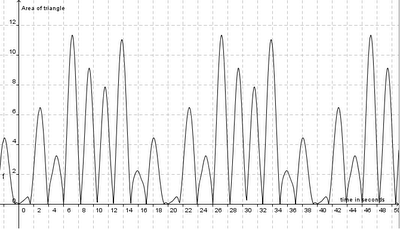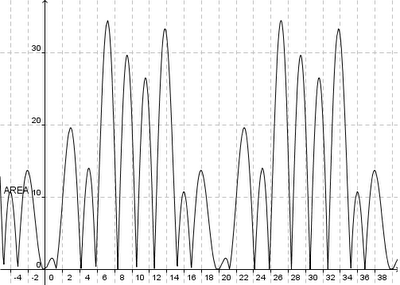## Friday, July 30, 2010

### Triangle Area - Confusing Sub-Periods!

I found a simplistic way to find the triangle area, that does not involve piecewise functions. You basically use the angle measured from the fixed vertex in your calculation of the area. The resulting equation is still somewhat hairy and spikey, but at least it's all in one piece.

Here is the demo that illustrates the moving triangle, with only one fixed point and two vertices rotating each at a different rate around the circle.

Screenshot (much less exciting than the animation linked to above):Graph of area over time. Notice that this is much more interesting, because you can see the times at which the two points overlap on the circle. NOTE: This graph is incorrect; corrected version is below in the Addendum!Now, here is my question after looking at this problem for a bit and feeling like it's driving me nuts: Why are there 9 periods between t = 0 and t = 20 (by examination of the graph)? Is there a mathematical way of figuring this out? (Is it simply that one point completes 4 cycles and the other completes 5 cycles in 20 seconds, so the sum is how many times both points are collinear with E?? If so, how can you justify that geometrically?)

ARGH. I must be thinking about this the roundabout way, and I hate that! If you can see through this fog, please enlighten me. Otherwise it'll keep driving me nuts.

--------------------

Addendum 7/31/10: Thanks for the catch, Matt! Here's the fixed graph.This time, I saved the file with all the helper functions for you to look at. I tried to name them descriptively, but ED(x) and EC(x) represent the lengths of two sides as a function of time, and thetaE(x) represents the angle at E as a function of time. AREA(x) is the final area calculated as a function of time, using Law of Sine. Cheers!

1.I noticed that the height of the graph is off in your graph of time vs. area. In the animation it is clear that the area has a maximum of around 32. I am close to a formula for the area, and I think some application of trig identities can help understanding what is going on in the graph.

2.argh! you're right! I'm gonna take a look at this again. I think it's a matter of adjusting some constants, because I 1.) verified the trig area formula I used by comparing it against GeoGebra's built-in polygon area calculation, and 2.) supposedly just pasted over the area formula again when I created the graph. Clearly I messed up somewhere in Step #2 and didn't notice it. Thanks for the sanity check! (I only visually toggled to check that max occured around t = 7.)

By the way, max area is more like 34 and occurs around t = 7. I'll have to take a look at this again. If you hover over the applet you can see the function I had intended to graph... I must have messed up when I transfered it over, because unfortunately I'm working off of my bf's computer (away from home right now) and didn't want to download a proper version of GeoGebra and/or save files properly. Doh!

Thanks again for watching my back :)
M

3.Cheers, Matt. I fixed it. Thanks again for the catch. :) :) Now I know I have one reader. Hah!

4.Mimi,

I am really enjoying this problem. I am using the fact that the area of triangle can be found by taking the half of the absolute value of the determinant with two of the columns being vectors that define two of the sides.

I think I will post some of it on my blog, and of course link it to yours.

5.I'm glad you like it! In general, how do you like the idea of 1. having kids look at a piece of mathematical animation and coming up with a modeling equation in order to analyze the motion and/or 2. having kids create to spec a piece of math animation on their own?

I'm just playing, but I'm interested in how this could be expanded to class.